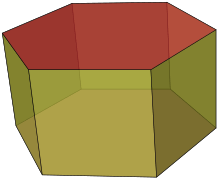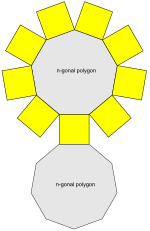Prism (geometry) facts for kids

Kids Encyclopedia Facts - Homework Help
Set of uniform prisms(A hexagonal prism is shown)
Type uniform polyhedron
Faces 2+n total:
2 {n}
n {4}
Edges 3n
Vertices 2n
Schläfli symbol {n}×{} or t{2, n}
Coxeter-Dynkin diagram Template:CDD
Vertex configuration 4.4.n
Symmetry group Dnh, [n,2], (*n22), order 4n
Rotation group Dn, [n,2]+, (n22), order 2n
Dual polyhedron bipyramids
Properties convex, semi-regular vertex-transitiven-gonal prism net (n = 9 here)

A Prism is a three-dimensional shape, which consists of two identical polygons, which do not lie in the same axis. For each side of the polygon, there will be a parallelogram.

Images for kidsPrism (geometry) Facts for Kids. Homework Help - Kiddle Encyclopedia.